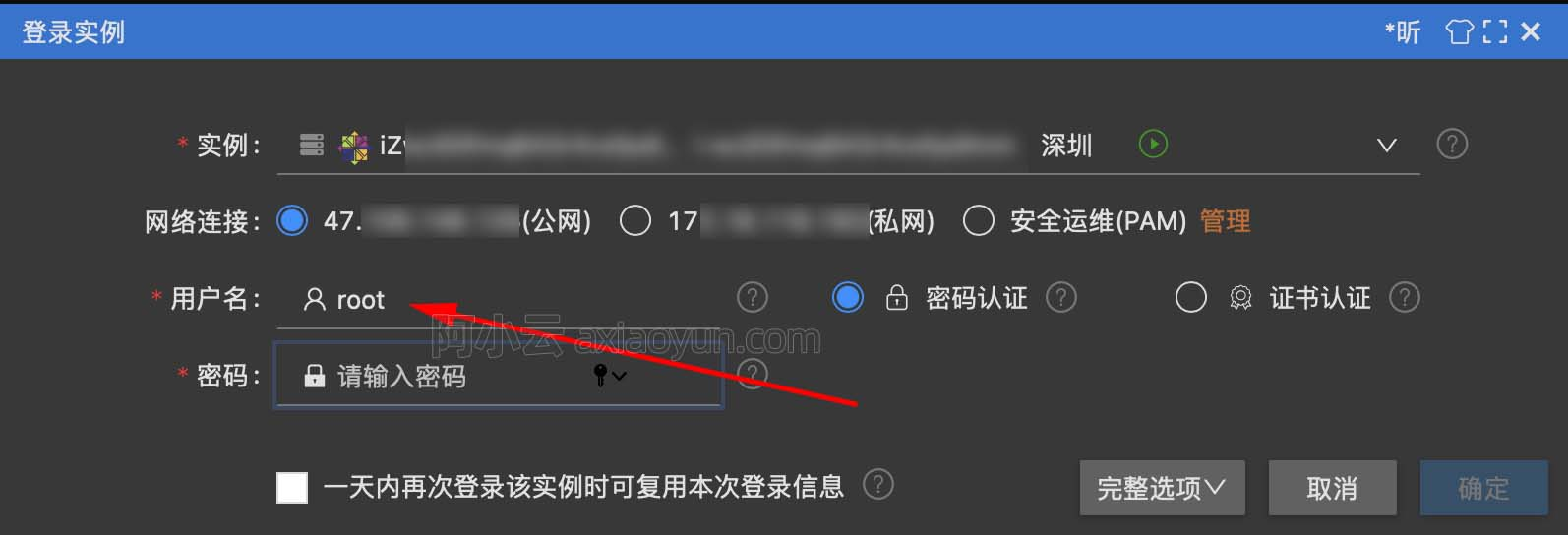# iOS程序猿如何快速掌握 PHP,化身&quot;全栈攻城狮&quot;?

+关注继续查看

## Hello World!

### 2.打开Atom编辑器,使用 cmd+N 新建文件,并输入以下代码,并 cmd+S 保存到 find_php目录,文件命名为 index.php.

<?php
echo 'Hello World';
?>


## AppDelegate 入口文件

iOS应用通常是以 AppDelegate 文件作为编码的起始(准确说的是 main.m,在此不细究).在PHP中,你可以使用一个 index.php 文件作为你的 php 程序的唯一入口.你的所有的php页面间的访问与跳转,都将是由此处开始.下面的代码可以先先复制到你的 index.php种,它实现了一个基本的页面访问与控制的框架:

<?php
$controller = '';$model = array();

if (isset($_GET['viewController'])) {$controller = $_GET['viewController']; } if (isset($_GET['model'])) {
$model =$_GET['model'];
}

echo '控制器:'.$controller.'<br />'; echo '数据模型:<br />'; foreach ($model as $key =>$value) {
echo $key.':'.$value.'<br />';
}

?>


控制器:HomeViewController

id:42
name:iOS122
age:25


viewController=后面跟的是你你的表示你的视图控制器, model是一个字典,用于存储数据模型,支持输入多个键值对.id,name,age等都是自定义的键,用于表示你想要传给新页面的数据,如果没有可以不写.

## MVC 设计模式

### 改进后的 index.php

<?php // index.php
/* 实现类文件的自动加载 */
function __autoload($className) { if (file_exists($className . '.php')) {
require_once $className . '.php'; return true; } return false; } // -------------------------------- /* 获取用户想要访问的页面的相关信息. */$controllerName  = '';
$model = array(); if (isset($_GET['viewController'])) {
$controllerName =$_GET['viewController'];
}

if (isset($_GET['model'])) {$model = $_GET['model']; } /* 跳转到指定页面. */ if ('' !==$controllerName) {
/* 我们约定每个控制器都至少有一个$model属性和 show方法 */$controller = new $controllerName();$controller->model = $model;$controller->show();
}
?>


• php 是一种弱类型语言,你定义变量时不必声明类型,但是变量要以 美元符号 $开头. • php 使用 new 函数来创建一个对象,语法是 new 类名(),这不禁让我想起 oc 中的 new函数,它的语法是: [类名 new]; • php 中的函数,看起来更像是C语言函数,也许说更像 oc 中的block,可能更好理解些. • php 访问属性,使用的是 ->,而不是 .;另一种 php 访问属性的方式是使用 obj['属性名'],如$controller['model'].

syntax error, unexpected '>' in /Applications/XAMPP/xamppfiles/htdocs/find_php/HomeViewController.php on line 38


### Controller:定义视图控制器

<?php // HomeViewController.php

/* 建议一个文件中只有一个 与文件同名的类.

class HomeViewController
{
/*
定义属性,允许定义时,给属性一个默认值,这一点比OC灵活.
public 关键字用于指定外部可访问;
类似的还有 private(仅允许内部访问),protected(仅允许自身及其子类访问);
属性前必须有关键字 public/private/protected 中的一个.
*/
public $model = array(); // 定义允许外部访问的属性. /* 构造函数,相当于init初始化方法; 当调用New 函数新建对象时,此方法会被自动调用; array 指明参数类型,$model是实参, $model = array(),用于指定默认参数; 指定了默认参数的参数,在调用时,可以不传; public 关键字作用等同于属性的关键字,默认可以不传,不传则为public; */ public function __construct(array$model = array())
{

/* 在实例方法内部访问对象的属性,使用 $this 关键字,且属性名前没有美元符号$;
类似于 oc 中的self,但是使用的是 -> 而不是 . */
$this->model =$model;
}

/*
析构函数,作用和 oc 中的dealloc很像.
*/
public function __destruct()
{
$this->model = NULL; } /* 获取内容,用于输出显示. */ protected function getContent() { /* 默认把用户输入以JSON格式返回 */$content = json_encode($this->model); return$content;
}

/*
定义实例方法:show;
定义方法使用的是关键字 function,且无法指定返回值,这点不如 oc 方便;
*/
public function show()
{
/* 使用$this关键字来调用另一个实例方法. */$content = $this->getContent(); echo$content;
}
}


{"id":"42","name":"iOS122","age":"25"}


### Model: 关于数据模型的几点说明.

• 网上关于MVC中M的讨论,此处我选取的是最基本的一种: M专指用于存储某种数据的类的实例.它可以用于数据的格式化存储和传递,但不应包含发起网络请求和读写数据库等操作;
• 在本文讨论的Model中,我们进一步简化了Model,允许且只允许用于通过URL来定义某个控制器的Model;
• PHP 是一本弱类型的语言,所以不必专门为某种控制器指定某种类型的Model.
• "PHP 中的数组实际上是一个有序映射。映射是一种把 values 关联到 keys 的类型。此类型在很多方面做了优化，因此可以把它当成真正的数组，或列表（向量），散列表（是映射的一种实现），字典，集合，栈，队列以及更多可能性。由于数组元素的值也可以是另一个数组，树形结构和多维数组也是允许的。"

### View: 一个显示HTML的实例.

  /* 获取内容,用于输出显示. */
protected function getContent()
{
$content = '<html><body><ul>'; foreach ($this->model as $key =>$value) {
$content .= "<li>$key:$value</li>"; }$content .= '</ul></body></html>';

return \$content;
}


• id:42
• name:iOS122
• age:25

<html>
<body>
<ul>
<li>id:42</li>
<li>name:iOS122</li>
<li>age:25</li>
</ul>
</body></html>


## 小结19764 0iOS程序猿如何快速掌握 PHP,化身&quot;全栈攻城狮&quot;?

1469 063945 029066 0iOS程序猿如何快速掌握 PHP,化身"全栈攻城狮"?

1100 022526 0Xshell使用SSH远程登录阿里云ECS服务器CentOS7
22305 016411 015936 020682 0

149

0

JS零基础入门教程（上册）